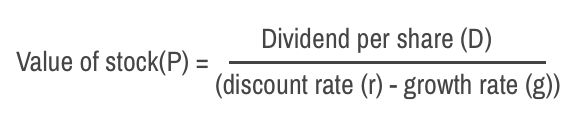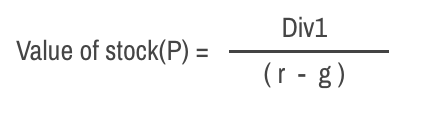# Stock Valuation: Dividend Discount Model (DDM)

Stock Valuation: Dividend Discount Model (DDM)

When you are investing for the long-term, it can be sensibly concluded that the only cash flow that you will receive from a publicly traded company will be the dividends, till you sell the stock.

Therefore, before investing it may be justifiable to calculate the dividends cash flow that you’ll receive while holding the stock. Dividend discount model (DDM) uses the same approach to find the worth of a stock.

In financial words, dividend discount model is a valuation method used to find the intrinsic value of a company by discounting the predicted dividends that the company will be giving (to its shareholders in future) to its present value.

Once, this value is calculated, it can be compared with the current market price of the stock to find whether the stock is overvalued or decently valued.

Don’t worry if you find this concept a little difficult to grasp right now. Read the post until the very end and this model will become crystal clear to you.

## What is Dividend Discount Model (DDM)?

Dividend discount model aims to find the intrinsic value of a stock by estimating the expected value of the cash flow it generates in future through dividends. This valuation model is derived from the net present value (NPV) and time value of money (TVM) concept.

Dividend discount model uses this simple formula:Here,

P= value of stock
D= Dividend per share
r= Discount rate (also known as required rate of return or cost of equity)
g= expected dividend growth rate.

Assumptions: While calculating the value of a stock using dividend discount model, the two big assumptions made are future dividend payments and growth rate.

Limitations: Dividend discount model (DDM) does not work for companies that do not provide dividends.

## Calculations

### A. Dividend growth rate (g):

The dividend growth rate (g) can be found using the company’s historical dividend growth.

Further, the dividend growth rate can also be calculated using return on equity (ROE) and retention rate values. Here’s a simple formula to calculate dividend growth rate:

Dividend growth rate = ROE * Retention rate

{Where, retention rate = (Net income – dividends)/ Net income = (1 – payout ratio) }

Therefore, Dividend growth rate = ROE * (1 – payout ratio)

ROE and payout ratio can be determined using company’s financial statements. An easier approach would be to refer financial websites like Money control, investing etc. You can find these values on most of the financial websites.

### B. Discount rate or Rate of return (r):

In the dividend discount model, if you want to get an annual return of 10% for your investment, then you should consider the rate of return (r) as 0.10 or 10%.

Further, r can also be calculated using Capital asset pricing model (CAPM). Under this model, the discount rate is equal to the sum of risk-free rate and risk premium. The risk premium is calculated as the difference between the market rate of return and the risk-free rate of return, multiplied by the beta.For example, for a company, if the beta is 1.5, the risk-free rate is 3% and the market rate of return is 7%.

Risk premium= ((7% – 3%)x1.5) = 6%.

Discount rate, r= risk free rate + risk premium = 3% + 6%= 9%

## TYPES OF DIVIDEND DISCOUNT MODELS

Now that you have understood the basics of Dividend Discount Model, let us move forward and learn three types of Dividend Discount Models.

1. Zero Growth Dividend Discount Model
2. Constant Growth Dividend Discount Model
3. Variable Growth Dividend Discount Model

## 1. Zero Growth Dividend Discount Model

The Zero growth dividend discount model assumes that all the dividends that are paid by the company remain same forever (until infinity).

Therefore, here the dividend growth rate (g) is zero.

As dividend is constant throughout the company life
The dividend is first year= dividend in second year = dividend in the third year…
Div1= Div2= Div3 = Div4 …. =Div

Here is the share value formula for the zero growth dividend discount model:Or Value of stock (P) = Div /r

Let us understand this further with the help of an example.

Example 1: Assume company ABC gives a constant annual dividend of Rs 1 per share till perpetuity (lasting forever). The required rate of return on the stock is 5%. Then what should be the purchasing price of the stock of company ABC?

Here, Expected return/ required rate of return (r) = 5%

Dividend (Div) = Rs 1 = Constant

Value of stock (P) = Div/r =1/0.05 = Rs 20.

Therefore, the purchasing price of the stock ABC should be less than Rs 20 to get the required rate of return of 5% per annum.

Limitations of Zero growth dividend discount model:

As the company grows bigger, it is expected to increase company’s dividend per share. The dividend cannot be constant till perpetuity.

## 2. Constant Growth Dividend Discount Model

This dividend discount model assumes that dividends grow at a fixed percentage annually. They are not variable and are constant throughout the life of the company. The most common model used in the constant growth dividend discount model is Gordon growth model (GGM)

### Gordon Growth Model (GGM):

The Gordon growth model for DCF is quite simple and straightforward. Here are the three values required to calculate the share value of a company:

• Div= Dividend at the zeroth year.
• r = company’s cost of capital/ required rate of return
• g=constant growth rate of dividends till perpetuity

Here, Div1= Dividend per share expected to be received at the end of first year = Div (1+g)

For the constant growth dividend discount model,Note: If you want to learn how the derive the above formula, you can find it here.

Let us solve an example to find the share price of a company with Gordon growth model.

Example 2: Assume a company QPR has a constant dividend growth rate of 4% per annum for perpetuity. This year the company has given a dividend of Rs 5 per share. Further, the required rate of return for the company is 10% per annum. Then, what should be the purchase price for a share of company QPR?

Here,

• Div= Dividend at zeroth year = Rs 5
• r = required rate of return = 10%
• g= constant growth rate of dividends till perpetuity= 4%

Div1= Dividend per share expected to be received at the end of first year = Div (1+g) = 5 (1+0.04) = Rs 5.2

Value of share (P) = Div1/ (r-g) = 5.2 / (0.1 -0.04) = 5.2/0.06 = Rs 86.67

Therefore, you should purchase the stock at a price below Rs 86.67 to get a required rate of return of 10% per annum.

### Limitations of Gordon Growth Model:

Here are few genuine limitations to Gordon growth model while performing constant growth dividend discount model

1. The constant growth rate for perpetuity is not valid for most of the companies. Moreover, Newer companies have fluctuating dividend growth rate in the initial years.
2. The calculation is sensitive to the inputs. Even a small change in the input assumption can greatly alter expected value of the share.
3. High growth problem. If the dividend growth rate becomes higher than the required rate of return i.e. g>r, then the value of the share price will become negative, which is not feasible.

## 3. The multi-level/Variable Growth Dividend Discount Model:

The multi-level growth rate for dividends model may divide the growth rate into two or three phases (according to the assumption).

In the two-stage growth rate DDM Model, the dividends grow at a high rate initially followed by a lower constant rate for later years. (Also read: Supernormal dividend growth model)

Further, in the three-stage growth DDM Model, the first stage will be a fast initial phase, then a slower transition phase an then ultimately ends with a lower rate for the infinite period. For example- A company XYZ’s dividend may grow at 5% rate for the first 7 years, 3% rate for the next 4 years and finally 2% rate in perpetuity.

If you are interested to read more, here is an amazing source to learn the multi-level growth rate dividend discount model.

Limitation:

The biggest drawback with the multi-level growth rate of dividend discount model is that’s it’s really difficult to assume the growth rate in small specific periods. There are a lot of uncertainties involved while making these assumptions when the growth is distributed at multiple levels.

## Problems of forecasting value using DDM:

Here are few of the common limitations of forecasting share value using the dividend discount model:

• Plenty of assumptions about the dividend growth and company’s future.
• This valuation model is good only as far the assumptions are good.
• Most of the inputs of DDM model keeps on changing and susceptible to error.
• Not feasible for few categories of stocks like Growth stocks. These stocks pay little or no dividends but rather use the company profit in their growth. DDM might never find these stock suitable for investment no matter how good the stock is.

## Conclusion:

Most of the analysts ignore dividend discount model while valuing the stock price because of its limitations as discussed above.

However, for few specific stocks (like stable dividend paying stocks), DDM remains a useful tool for evaluating stocks. Dividend discount model is a simple and straightforward method of stock valuation. It demonstrates how the stock value can be calculated by a simple approach of discounting future cash flows.

Anyways, I will highly recommend to never invest in a stock based on just DDM valuation. Use other financial tools like ROE, PE etc to cross-verify the conclusions before investing.

In the end, I would like to add that although DDM is criticised for the limited use, however, it has proved useful in the past. In addition, here is an amazing quote regarding valuation by one of the greatest investor of all time, Warren Buffett.

“It is better to be approximately right than precisely wrong.” -Warren Buffett